# Solution October 3, 2007

### Problem

The sequence {an} is defined by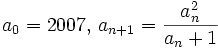$a_{0}= 2007,\, a_{n+1}=\frac{a_{n}^{2}}{a_{n}+1}$ for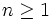$n \ge 1$. Prove that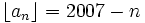$\lfloor a_{n}\rfloor =2007-n$ for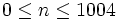$0 \le n \le 1004$, where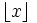$\lfloor x\rfloor$ denotes the largest integer no larger than x.

### Solution

The recurrence is equivalent to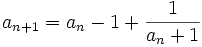$a_{n+1} = a_n-1 + \frac1{a_n+1}$

so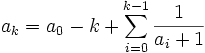$a_{k} = a_0 - k + \sum_{i=0}^{k-1} \frac1{a_i+1}$

Note that$a_{k} \geq a_0 - k = 2007 - k$. Therefore we have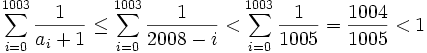$\sum_{i=0}^{1003} \frac1{a_i+1} \leq \sum_{i=0}^{1003} \frac1{2008-i} < \sum_{i=0}^{1003} \frac1{1005} = \frac{1004}{1005} < 1$

Also,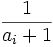$\frac1{a_i+1}$ is positive for i < 1004. Thus,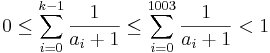$0 \leq \sum_{i=0}^{k-1} \frac1{a_i+1} \leq \sum_{i=0}^{1003} \frac1{a_i+1} < 1$

for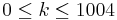$0\leq k \leq 1004$. Thus,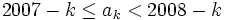$2007 - k \leq a_{k} < 2008 - k$

which implies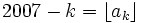$2007 - k = \lfloor a_{k} \rfloor$, completing the proof.